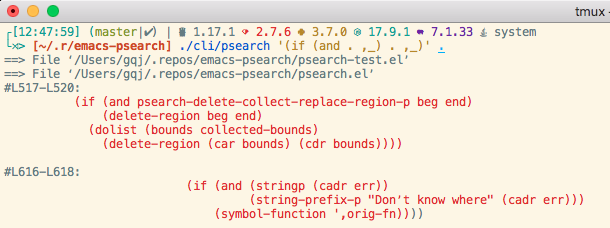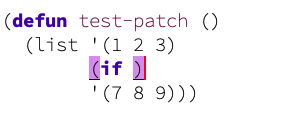# psearch: 基于 pcase 的 elisp 代码搜索工具

5 个赞

`pcase-refactor-at-point` 有个非常明显的缺点，就是光标必须位于 sexp 头部

• `|(sexp)`• `(se|xp)```````(defun psearch-refactor-setq-local-at-point ()
(interactive)
(psearch-search-at-point '`(set (make-local-variable ',sym) . ,val)
'`(setq-local ,sym ,@val)))

;;        修改前                        修改后
;;    |(match a (b c))     =>     |(replace a (b c))
;;     (match a (b|c))     =>     |(replace a (b c))
``````

``````(let ((a 1)
(b (1+ a|)))
...)
``````

``````(defun psearch-refactor-combine-setq-in-region (beg end)
(interactive "r")
(save-excursion
(save-restriction
(narrow-to-region beg end)
(goto-char (point-max))
(unwind-protect
(let ((sym-val-list nil))
(while (psearch-backward
'`(setq ,sym ,val)
'`(,sym ,val)
(lambda (result _bounds)
(setq sym-val-list (append result sym-val-list)))))
(delete-region beg end)
(insert (psearch--print-to-string `(setq ,@sym-val-list))))))))
``````

``````(defun psearch-refactor-split-setq-at-point ()
(interactive)
(psearch-replace-at-point '`(setq . ,(and r (guard (> (length r) 2))))
'(->> (psearch--print-to-string
(mapcar
(lambda (pair)
(cons 'setq pair))
(seq-partition r 2)))
(string-remove-prefix "(")
(string-remove-suffix ")"))))
``````

EDIT: 其实可以直接在 pattern 内部完成去 `()` 的步骤（原先使用的 :filter 关键字已不再提供），`el-search` 应该也一样。不过这样会使得 pattern 变臃肿。

```````(setq ,s1 ,v1 ,s2 ,v2) -> `((setq ,s1 ,v1) (setq ,s2 ,v2))
``````

C-h f el-search-query-replace

It is possible to replace matches with an arbitrary number of expressions (even with zero expressions, effectively deleting matches) by using the “splicing” submode that can be toggled from the prompt with “s”. When splicing mode is on (default off), the replacement expression must evaluate to a list, and all of the list’s elements are inserted in order.

1 个赞

EDIT1: 暂且通过增加一个 `collect` 概念来实现合并操作吧。

``````(psearch-replace '`(setq ,sym ,val)
;;  collect   ->  replace (at the end)
'(`(,sym ,val) `(setq ,@(-flattern-n 1 its))))
``````

EDIT2: 引入 collect 带来的新问题：如果每个匹配项之间不是连续的，就不能删除 (beg end) 之间的整块空间，只能删除每个匹配项的 bounds 区域。面临几种选择：

1. 删除 (beg end) 之间的内容，不考虑非连续的情况
2. 支持不连续的场景，只删除 bounds 区域，但也留下删除后的大量空洞。
3. 给个 custom 变量，让用户决定 1 还是 2。

`,@` 就是 `unquote-splicing`

1 个赞

el-patch 更简便易用，没那么多复杂的概念，心智负担更小，只要会有用 pcase 就能理解。

2 个赞文件多了速度有点慢。

``````(psearch-with-function-patch test-patch
(psearch-replace '`(if nil ,body)
'`(if t ,body)))
;; (defun test-patch ()         =>    (defun test-patch ()
;;   (list '(1 2 3)                     (list '(1 2 3)
;;         (if nil '(4 5 6))                  (if t '(4 5 6))
;;         '(7 8 9)))                         '(7 8 9)))
``````

1. 对象函数：
``````(defun test-patch ()
(list '(1 2 3)
(if t '(4 5 6))
'(7 8 9)))
``````
1. 执行替换
``````(psearch-replace '`(if t ,body) '`(if nil ,body))
``````
1. 替换结果及光标位置：1. 缩进问题：Wrong indent for :bind · Issue #887 · jwiegley/use-package · GitHub
2. 参考方案：indentation - How to indent keywords aligned? - Emacs Stack Exchange （最高赞回答，回答的作者表示要给上游发补丁，但一直没打？）
``````(with-eval-after-load 'lisp-mode
(require 'psearch)
(psearch-patch calculate-lisp-indent
(psearch-replace
'`(if (or ,or1 ,_) ,then ,else)
'`(if (or ,or1
;; First sexp after `containing-sexp' is a keyword. This
;; condition is more debatable. It's so that I can have
;; unquoted plists in macros. It assumes that you won't
;; make a function whose name is a keyword.
(when-let (char-after (char-after (1+ containing-sexp)))
(char-equal char-after ?:))

;; Check for quotes or backquotes around.
(let* ((positions (elt state 9))
(last (car (last positions)))
(rest (reverse (butlast positions)))
(any-quoted-p nil)
(point nil))
(or
(when-let (char (char-before last))
(or (char-equal char ?')
(char-equal char ?`)))
(progn
(while (and rest (not any-quoted-p))
(setq point (pop rest))
(setq any-quoted-p
(or
(when-let (char (char-before point))
(or (char-equal char ?')
(char-equal char ?`)))
(save-excursion
(goto-char (1+ point))
(looking-at-p
"\\(?:back\\)?quote[\t\n\f\s]+(")))))
any-quoted-p))))
(null t) ;; 这儿本应使用 ",then", 但此处 ",then" 值为 nil,
;; 而 psearch 对应的 nil 功能是删除该匹配，所以用
;; (null t) 替代
,else))))
``````

```````(:token ,token
:token-quality ,quality)  ,
``````
``````(use-package org
:bind (:map org-mode-map
("C-c C-'" . org-edit-special)
:map org-src-mode-map
("C-c C-'" . org-edit-src-exit)))
``````

```````(:token ,token
:token-quality ,quality) .
``````
``````(use-package org
:bind (:map org-mode-map
("C-c C-'" . org-edit-special)
:map org-src-mode-map
("C-c C-'" . org-edit-src-exit)))
``````

psearch-patch 目前版本的副作用是，打完补丁后的原函数的位置找不到了:

``````(symbol-file 'calculate-lisp-indent)
;; >> nil
``````

1 个赞

psearch 是真的好用啊，无痛打补丁，打了好几个复杂的功能了，感谢大佬